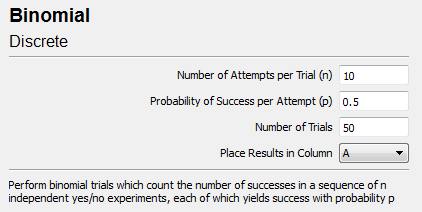﻿ Binomial

# Binomial

Top  Previous  NextThe binomial data generator allows you to simulate binomial trials.  A example problem that this distribution might simulate is: Roll a standard die ten times and count the number of sixes. The distribution of this random number is a binomial distribution with n = 10 and p = 1/6.